# Data @ Reed

Analyzing & Visualizing Data > Data Visualization with ggplot2

# Intro toggplot2

ggplot2 is an R package for data visualization that implements a common “Grammar of Graphics”. Here is an example of visualizing data, using ggplot2, creatinga scatterplot of some data from the built-in iris dataset.

library(ggplot2)

ggplot(iris, aes(x = Petal.Length, y = Sepal.Length, col = Species)) +
geom_point()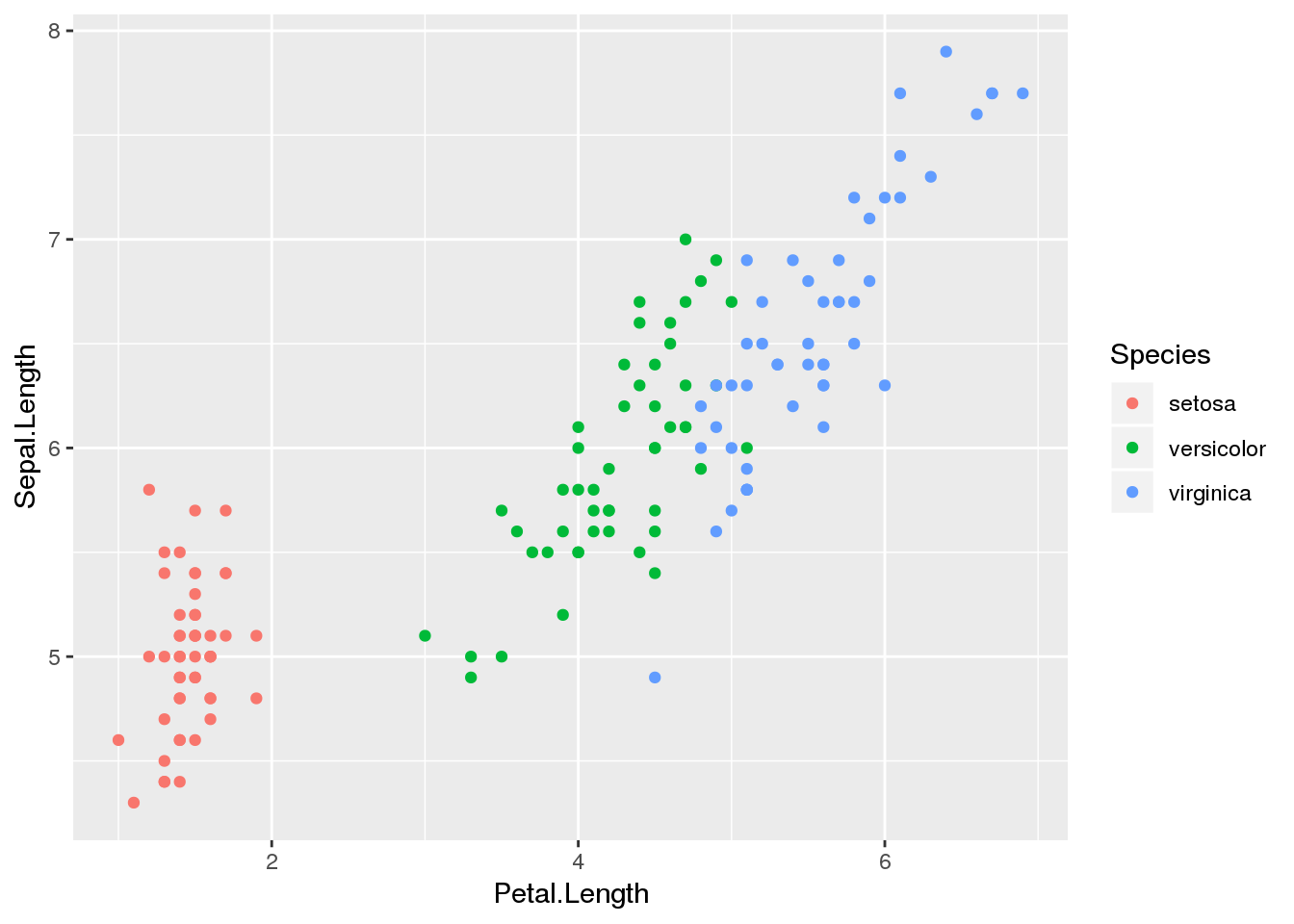The three most important parts of making any graph with ggplot are data, mappings, and layers, with the general template ggplot(data, aes(mappings)) + layers().

### Quick plots withqplot

If you already have a dataset ready and want a basic plot, the qplot function can help as a shortcut to ggplot. The arguments to qplot are less complicated than the full ggplot syntax, so you have fewer but simpler options.

qplot(data = iris, x = Petal.Length, y = Sepal.Length, geom = "point")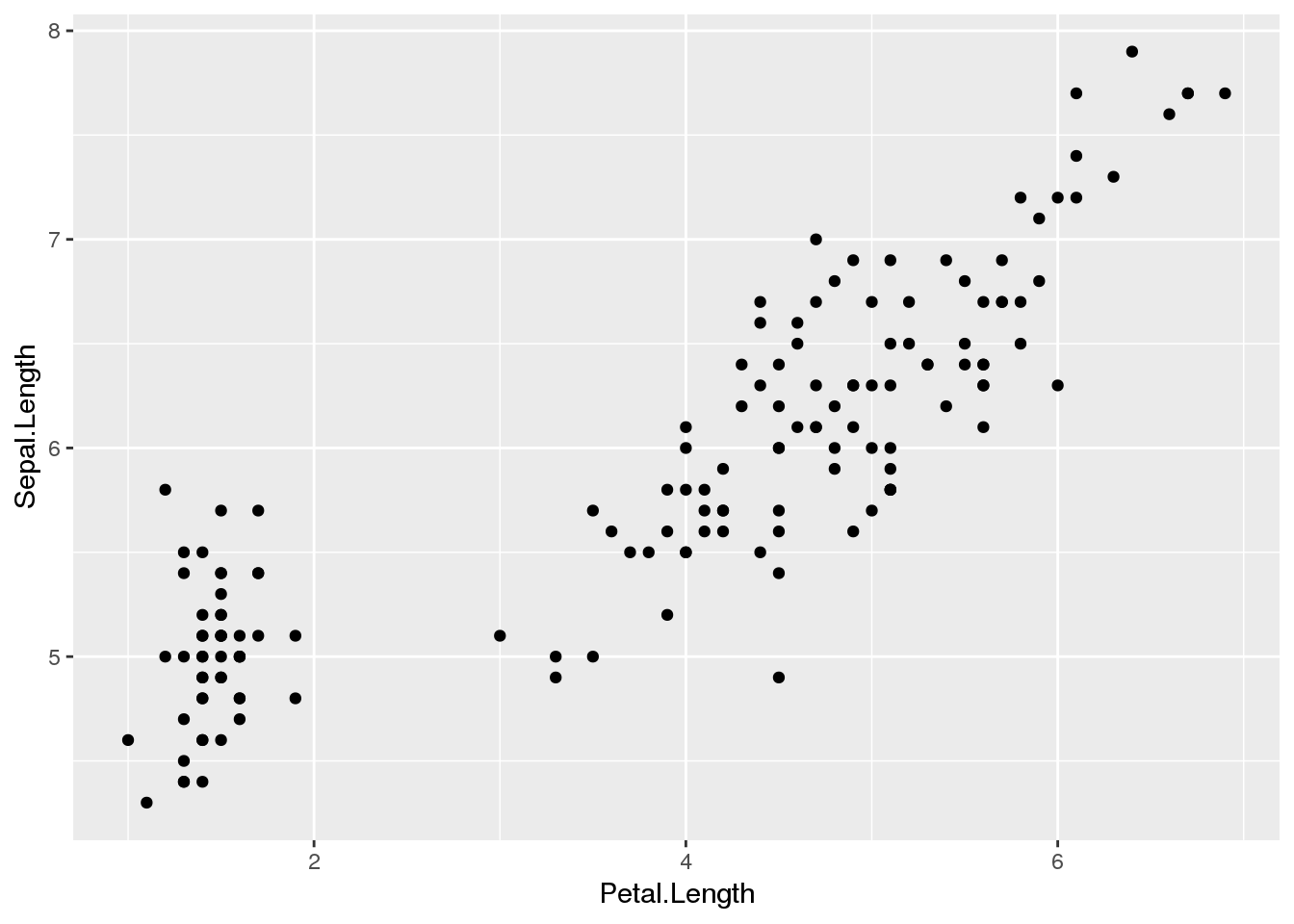This makes a scatterplot (geom = "point") of sepal length vs. petal length. To generate a histogram of sepal width for all flowers, use the following qplot command:

qplot(data = iris, x = Sepal.Width, geom = "histogram")
## stat_bin() using bins = 30. Pick better value with binwidth.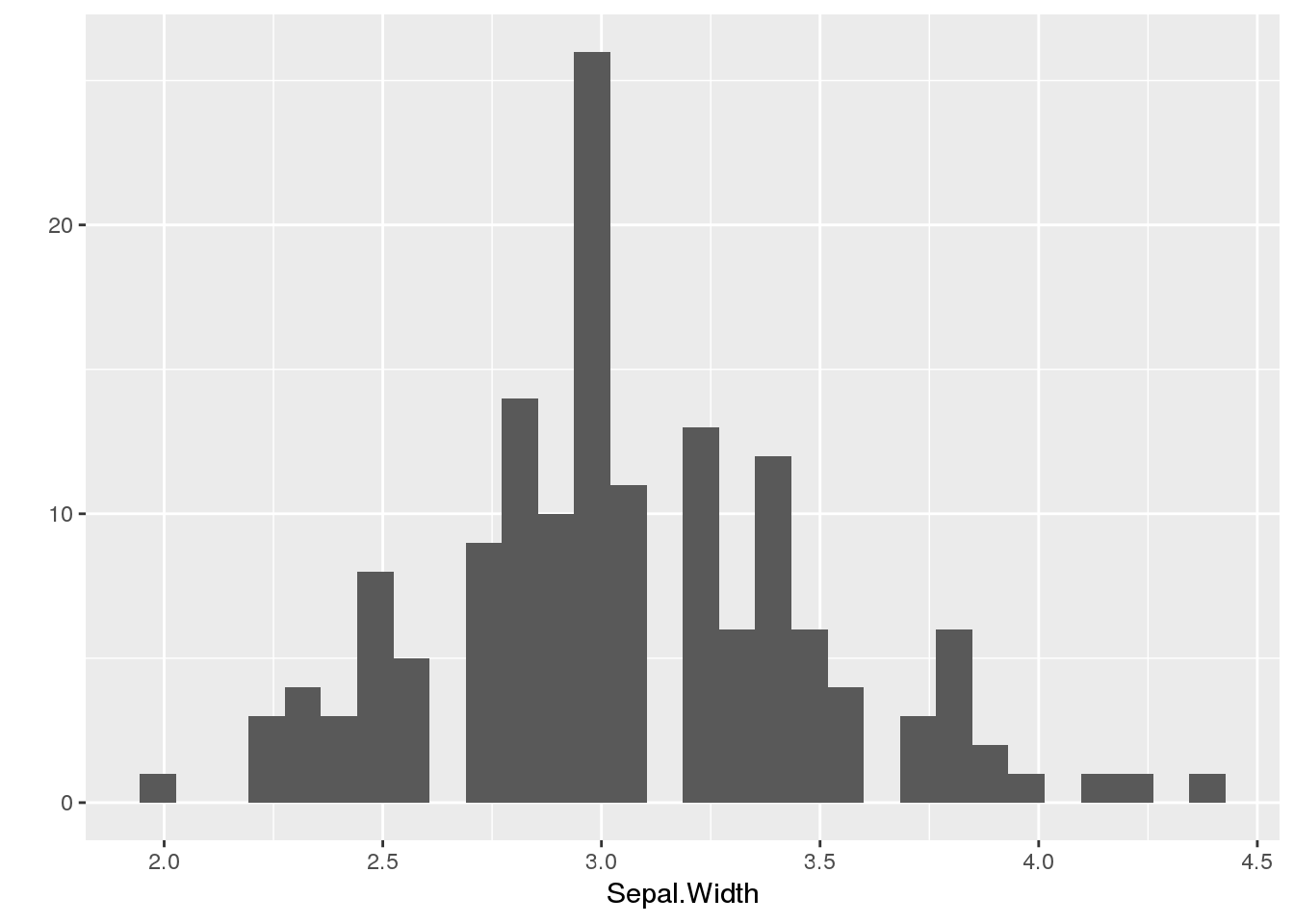### Data (and data structure)

The data that goes into ggplot should be a dataframe. (If you use another data structure, the function will try to convert your data into a data frame and possibly error out.)

When using ggplot2, each row in the dataset will be read as a data point, so make sure that the single rows in the data represent a single data point. (For more on this "tidy data" structure, see here).

If you want one row to represent two markers in the plot, you need to structure your data so that the row is split into two units. For example, let’s say you have the following data set of GDP growth (data from the World Bank).

Table of GDP data by country, across years. Values in columns 2-5 represent GDP of a country for a given year (2007-2010)
country 2007 2008 2009 2010
China 14.2 9.7 9.4 10.6
India 9.8 3.9 8.5 10.3
United States 1.8 -0.3 -2.8 2.5
Indonesia 6.3 6.0 4.6 6.2
Brazil 6.1 5.1 -0.1 7.5
Pakistan 4.8 1.7 2.8 1.6
Nigeria 6.8 6.3 6.9 7.8
Russia 8.5 5.2 -7.8 4.5
Mexico 2.3 1.1 -5.3 5.1

Using this dataset, ggplot can only print each row (country) as one data point.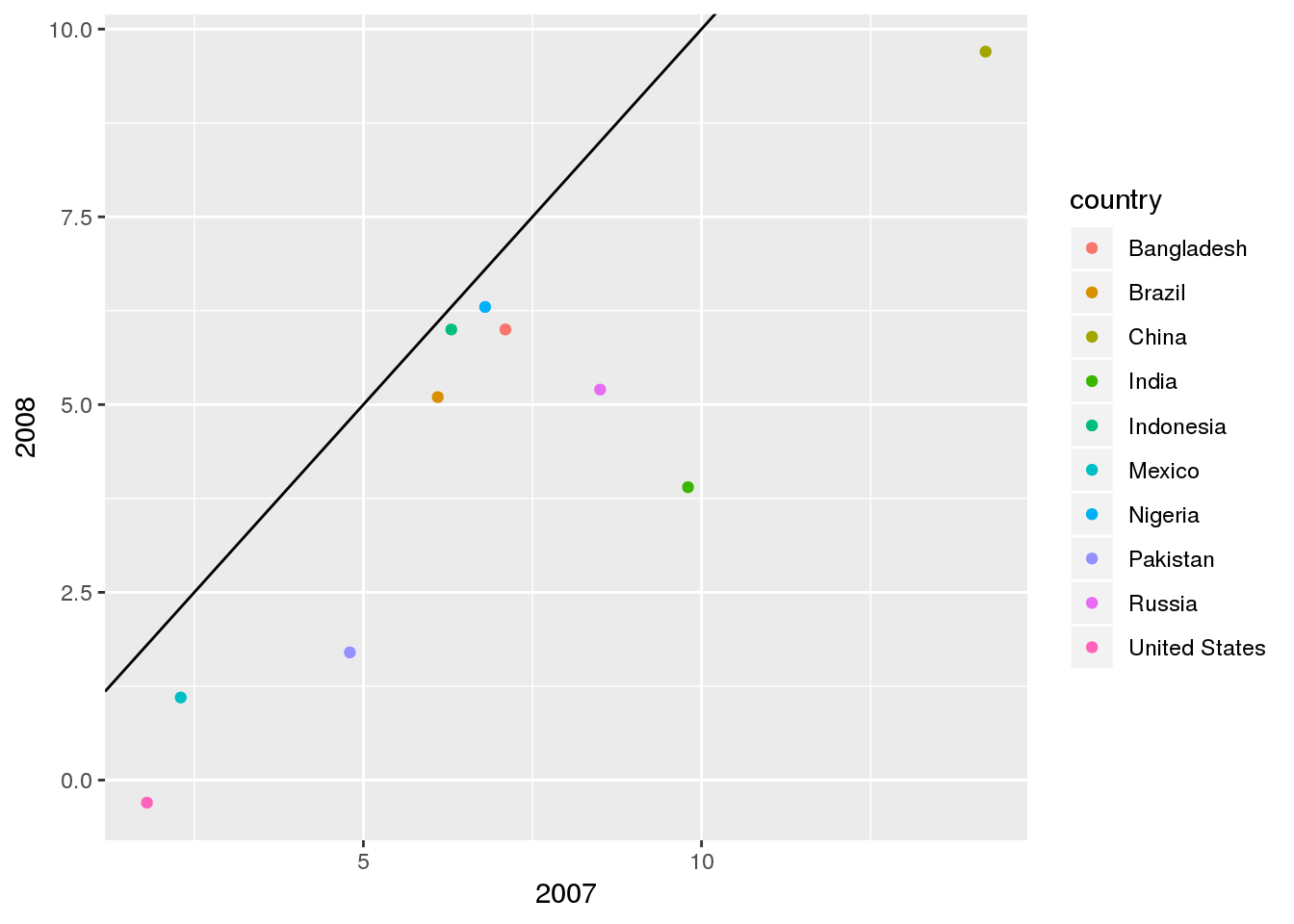The diagonal line is at $y=x$, which represents the same amount of growth in 2007 and 2008. Since all of the countries fall below this line, all countries had lower growth in 2008 than in 2007, but this is tricky to interpret. This may be easier to read if you have each country and year be a point, to directly see how the country changed over time.

gdp_tall <- tidyr::gather(gdp, key = year, value = growth, 2:5)
knitr::kable(head(gdp_tall))
Table of GDP data by country and year. Columns are (L to R) country name, year, and GDP.
country year growth
China 2007 14.2
India 2007 9.8
United States 2007 1.8
Indonesia 2007 6.3
Brazil 2007 6.1
Pakistan 2007 4.8

This is the first few rows of a dataset that places every country-year pair on a separate row. The tidyr package is a commonly-used tool for transforming data and creating new arrangements of the same information. With this new data setup, you can make a more informative graphic:

ggplot(gdp_tall, aes(x = year, y = growth, col = country)) +
geom_point() +
geom_line(aes(group = country)) +
labs(title = "GDP Growth by Year",
x = "Year",
y = "Growth (%)",
col = "Country")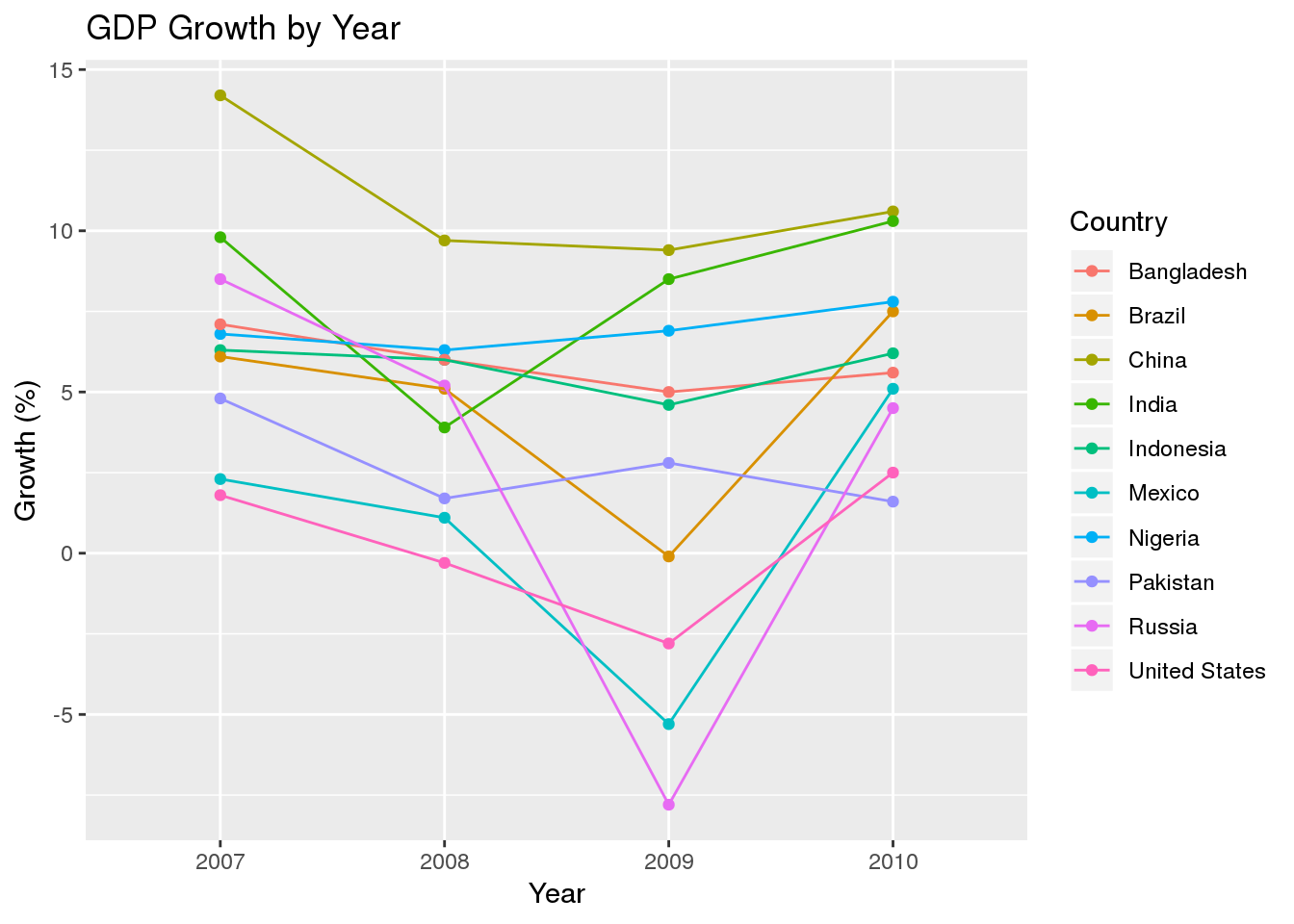In the above graphic, it is much more clear that GDP growth decreased across all countries in 2008, there was a mix of results in 2009, and in 2010 most of these countries had higher GDP growth. It is important to consider data formatting when plotting your information

### Mappings

Mappings turn data into visual differences. The earlier plot example with iris mapped petal length onto the x axis, sepal length onto the y axis, and species onto color. Thus each data point with a different petal length will have a different horizontal position, each with a different sepal length will have a different vertical position, and each with a different species will have a different color.

ggplot(iris, aes(x = Petal.Length, y = Sepal.Length, col = Species)) +
geom_point()

These visual cues are often caled “aesthetics”, and this word is where the aes()command comes from. Any mapping that takes a variable in the data to a visual aesthetic must go inside of aes(). Anything outside of that gets taken as an instruction for the entire plot to follow. (For example, specifying color = "red" outside of aes() will turn all of the data points red.)

The visual aesthetics that you will most often see are

• x

• y

• color

• size

• linetype (solid, dashed, dotted, etc.)

• alpha (transparency)

• fill (color for the inside of a region)

• shape

### Layers

Layers are the elements that plot the data. If you forget to add a layer, R will  create an empty plot. After defining your data and aesthetics inside the ggplot command, you can add on layers by separating each one with a +.

ggplot(gdp_tall, aes(x = year, y = growth, col = country)) +
geom_point() +
geom_line(aes(group = country)) +
labs(title = "GDP Growth by Year",
x = "Year",
y = "Growth (%)",
col = "Country")

The layers added in this plot earlier were:

• geom_point(), to add a dot for every row
• geom_line(), to connect these dots
• labs(), to edit the labels

You can put different aesthetic mappings into different layers as you need. Any aesthetic in the original ggplot() command will apply to all layers, but anything inside a single layer will only apply to that one. Above, the group aesthetic is placed inside of geom_line(), to tell R that the lines should connect points that have the same country.

### Other elements

There are many other elements to consider while using ggplot2, including themes, faceting, and labels. Check out the ggplot2 cheat sheet and the package website for more information on these features.